# tensorly.parafac2_tensor.parafac2_to_slices

parafac2_to_slices(parafac2_tensor, validate=True)[source]

Generate all slices along the first mode from a PARAFAC2 tensor.

Generates a list of all slices from a PARAFAC2 tensor. A list is returned since the tensor might have varying size along the second mode. To return a tensor, see the parafac2_to_tensor function instead.shape

The decomposition is on the form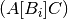such that the i-th frontal slice,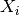, of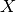is given by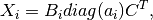where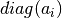is the diagonal matrix whose nonzero entries are equal to the-th row of the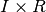factor matrix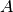,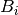is a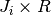factor matrix such that the cross product matrix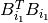is constant for all, and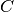is a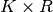factor matrix. To compute this decomposition, we reformulate the expression forsuch that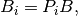where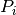is aorthogonal matrix and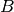is a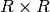matrix.

An alternative formulation of the PARAFAC2 decomposition is that the tensor element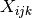is given by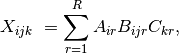with the same constraints hold foras above.

Parameters: parafac2_tensorParafac2Tensor - (weight, factors, projection_matrices) weights1D array of shape (rank, )weights of the factors factorsList of factors of the PARAFAC2 decompositionContains the matrices,anddescribed above projection_matricesList of projection matrices used to create evolvingfactors. List[ndarray]A list of full tensors of shapes [P[i].shape, C.shape], where P is the projection matrices and C is the last factor matrix of the Parafac2Tensor.